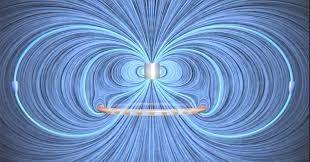electromagnetism代写电磁学代写

## RADIATION

Radiation: Electromagnetic fields that carry energy off to infinity.

At large distances, $\vec{E}$ and $\vec{B}$ fall off only as $1 / r$, so the Poynting vector falls off as $1 / r^{2}$. If the Poynting vector is then integrated over a large sphere, of area $4 \pi r^{2}$, the contribution approaches a constant as $r \rightarrow \infty$.

Recall the Liénard-Wiechert potentials:
$$\begin{array}{|c|} \hline V(\vec{r}, t)=\frac{1}{4 \pi \epsilon_{0}} \frac{q}{\left|\vec{r}-\vec{r}{p}\right|\left(1-\frac{\vec{v}{p}}{c} \cdot \hat{\boldsymbol{\kappa}}\right)} \ \vec{A}(\vec{r}, t)=\frac{\mu_{0}}{4 \pi} \frac{q \vec{v}{p}}{\left|\vec{r}-\vec{r}{p}\right|\left(1-\frac{\vec{v}{p}}{c} \cdot \hat{\boldsymbol{n}}\right)}=\frac{\vec{v}{p}}{c^{2}} V(\vec{r}, t) \end{array}$$
where $\vec{r}{p}$ and $\vec{v}{p}$ are the position and velocity of the particle at $t_{r}$
$$t_{r}=t-\frac{\left|\vec{r}-\vec{r}{p}\right|}{c}$$ and $$\hat{\boldsymbol{\kappa}}=\frac{\vec{r}-\vec{r}{p}}{\left|\vec{r}-\vec{r}{p}\right|}, \quad \boldsymbol{r}=\left|\vec{r}-\vec{r}{p}\right| .$$

## Approximation 1: $d \ll r$.

\begin{aligned} \boldsymbol{r}{\pm} &=\sqrt{r^{2} \mp r d \cos \theta+(d / 2)^{2}} \ & \Rightarrow\left{\begin{array}{l}\frac{1}{\boldsymbol{n}{\pm}} \simeq \frac{1}{r}\left(1 \pm \frac{d}{2 r} \cos \theta\right) \ \cos \left[\omega\left(t-\boldsymbol{r}_{\pm} / c\right)\right] \simeq \cos \left[\omega(t-r / c) \pm \frac{\omega d}{2 c} \cos \theta\right]\end{array}\right.\end{aligned}
$d \ll r$ is ALWAYS valid for radiation, which is defined in the $r \rightarrow \infty$ limit.

## Approximation 2: $d \ll \frac{c}{\omega}$.

Since $\lambda=2 c / \omega$, this is equivalent to $d \ll \lambda$. This is the IDEAL DIPOLE APPROXIMATION. This is really the first term in a power expansion in $d / \lambda$, the multipole expansion for radiation, but we will go no further than the dipole. Implies
$$\cos \left[\omega\left(t-\boldsymbol{n}{\pm} / c\right)\right] \simeq \cos [\omega(t-r / c)] \mp \frac{\omega d}{2 c} \cos \theta \sin [\omega(t-r / c)]$$ Then, defining $p{0}=q_{0} d$,
$$V(r, \theta, t)=\frac{p_{0} \cos \theta}{4 \pi \epsilon_{0} r}\left{\frac{1}{r} \cos [\omega(t-r / c)]-\frac{\omega}{c} \sin [\omega(t-r / c)]\right}$$

## Approximation 3: $r \gg \lambda$.

The region $r \gg \lambda$ is called the radiation zone. This approximation is ALWAYS valid for discussing radiation. Implies that the first term in curly brackets can be dropped:
$$V(r, \theta, t)=-\frac{p_{0} \omega}{4 \pi \epsilon_{0} c}\left(\frac{\cos \theta}{r}\right) \sin [\omega(t-r / c)]$$
Summary of approximations: $d \ll \lambda \ll r$

Need also $\vec{A}$, which is due to the current in the wire. Recall
$$\vec{A}(\vec{r}, t)=\frac{1}{4 \pi \epsilon_{0}} \int \mathrm{d}^{3} x^{\prime} \frac{\vec{J}\left(\vec{r}^{\prime}, t_{r}\right)}{\left|\vec{r}-\vec{r}^{\prime}\right|}$$
Here
$$\mathrm{d}^{3} x^{\prime} \vec{J}=I \mathrm{~d} \vec{\ell}=\frac{\mathrm{d} q}{\mathrm{~d} t} \mathrm{~d} z \hat{z}$$
$\mathrm{SO}$
\begin{aligned} \vec{A}(\vec{r}, t) &=\frac{\mu_{0}}{4} \int_{-d / 2}^{d / 2} \frac{-q_{0} \omega \sin [\omega(t-\boldsymbol{n} / c)]}{\boldsymbol{r}} \mathrm{d} z \ &=-\frac{\mu_{0} p_{0} \omega}{4} \sin [\omega(t-r / c)] \hat{z} \end{aligned}

Differentiating,
$$\begin{array}{c} \vec{E}=-\vec{\nabla} V-\frac{\partial \vec{A}}{\partial t}=-\frac{\mu_{0} p_{0} \omega^{2}}{4 \pi}\left(\frac{\sin \theta}{r}\right) \cos [\omega(t-r / c)] \hat{\theta} . \ \vec{B}(\vec{r}, t)=\frac{1}{c} \hat{r} \times \vec{E}(\vec{r}, t) . \end{array}$$

## Radiation Reaction

If an accelerating particle radiates energy, it must lose energy obviously! But it is not clear exactly how. Point charges and conservation laws cannot be combined rigorously.

For a nonrelativistic particle, radiation power is given by the Larmor formula:
$$P=\frac{\mu_{0} q^{2} a^{2}}{6 \pi c}$$
Maybe then,
$$\vec{F}{\mathrm{rad}} \cdot \vec{v} \stackrel{?}{=}-\frac{\mu{0} q^{2} a^{2}}{6 \pi c}$$
But not necessarily, since $P_{\text {Larmor }}$ represents the power destined to become radiation. There can be other energy exchanges with the near fields. Since the RHS of Eq. (34) does not depend on $\vec{v}$, there is no way to match the two sides.

For cyclic motion, the total energy loss over one cycle should match the energy loss described by the Larmor formula. If a cycle extends from $t_{1}$ to $t_{2}$ then
$$\int_{t_{1}}^{t_{2}} a^{2} \mathrm{~d} t=\int \frac{\mathrm{d} \vec{v}}{\mathrm{~d} t} \cdot \frac{\mathrm{d} \vec{v}}{\mathrm{~d} t} \mathrm{~d} t=\underbrace{\left.\left(\vec{v} \cdot \frac{\mathrm{d} \vec{v}}{\mathrm{~d} t}\right)\right|{t{1}} ^{t_{2}}}{=0}-\int{t_{1}}^{t_{2}} \frac{\mathrm{d}^{2} \vec{v}}{\mathrm{~d} t^{2}} \cdot \vec{v} \mathrm{~d} t .$$
So, energy conservation holds if
$$\int_{t_{1}}^{t_{2}}\left(\vec{F}{\mathrm{rad}}-\frac{\mu{0} q^{2}}{6 \pi c} \dot{\vec{a}}\right) \cdot \vec{v} \mathrm{~d} t=0$$
which will hold if
$$\vec{F}{\mathrm{rad}}=\frac{\mu{0} q^{2}}{6 \pi c} \dot{\vec{a}}$$

$$\vec{F}{\mathrm{rad}}=\frac{\mu{0} q^{2}}{6 \pi c} \dot{\vec{a}}$$
is the Abraham-Lorentz formula for the radiation reaction force. We have not proven it, but we have shown that it provides a force that is consistent with conservation of energy, at least for periodic motion.

The Abraham-Lorentz formula can be “derived” by modeling the point particle as an extended object, so that one can calculate the force that one part of the object exerts on the other parts. One then takes a limit in which the size of the extended object approaches zero. But the result depends on the details of the model, so the result remains inconclusive.路径积分物理代写认准UpriviateTA

BS equation代写